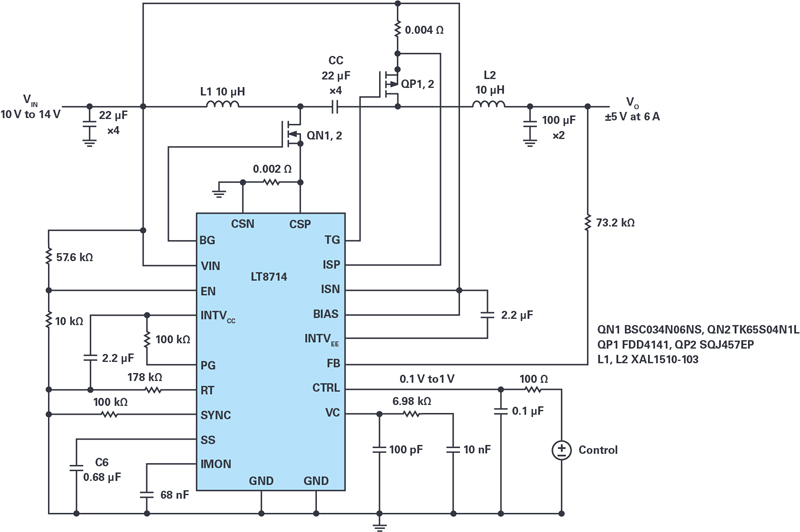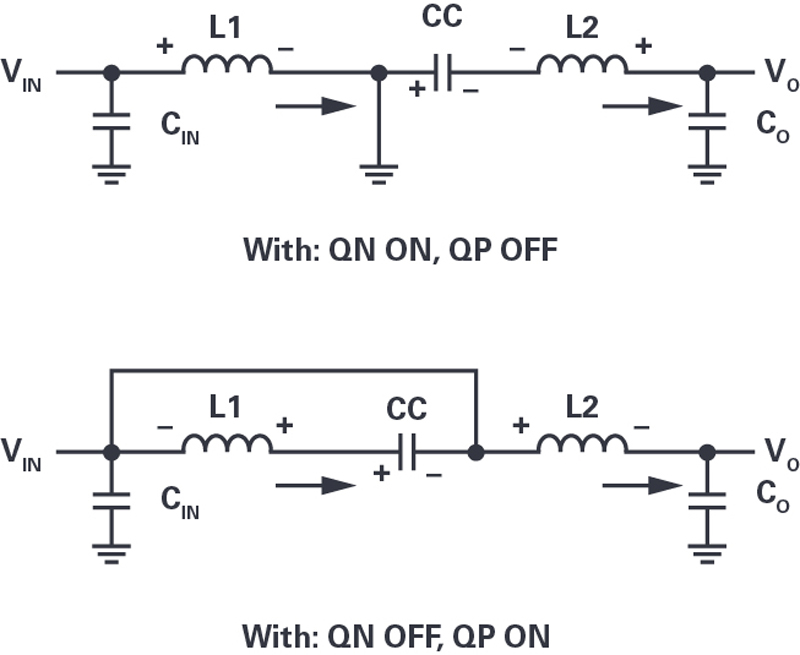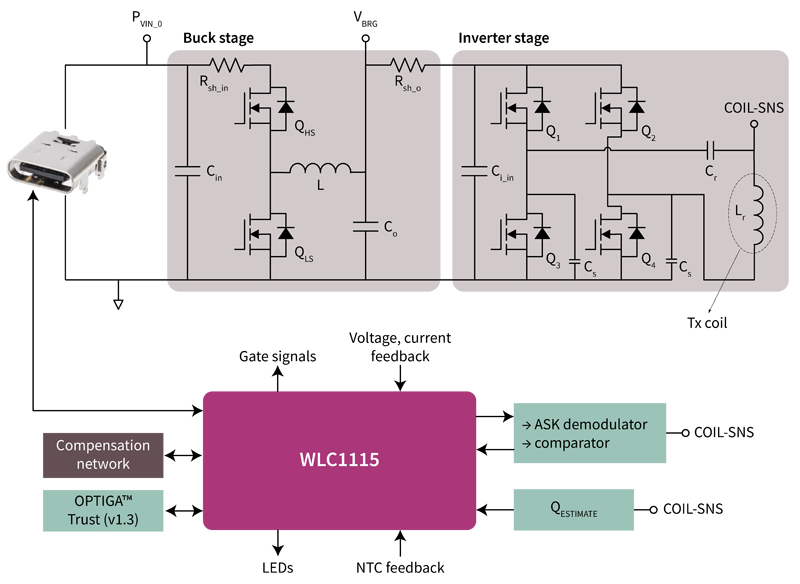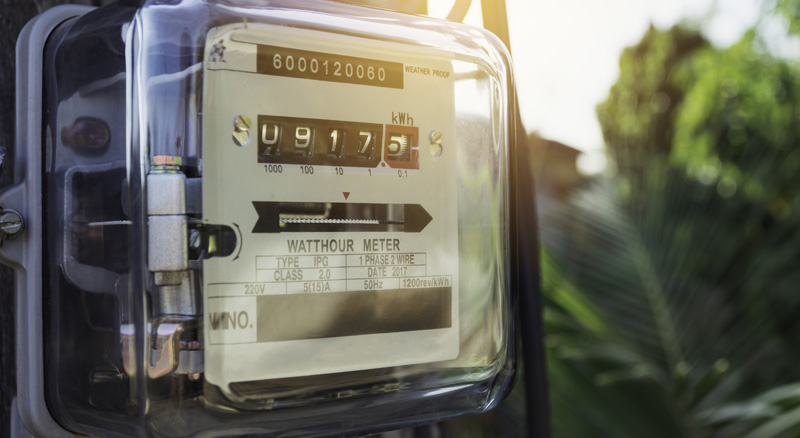# Two Terminal Bipolar Power Supply

Author:
Victor Khasiev, Senior Applications Engineer, Analog Devices

Date
05/02/2022PDFClick image to enlarge

Figure 1. An electrical schematic of a power supply based on the LT8714 operating in two quadrants VIN 12 V, VO ±5 V at 6 A

­A 2-quadrant power supply — one that provides a positive or negative voltage to the same output terminals — can easily be produced using the LT8714 4-quadrant controller. The 2-quadrant supply shown here can be used in a variety of applications ranging from window tinting — where changing polarity changes the alignment of crystal molecules — to test and measurement equipment.

The LT8714 data sheet describes the operation of the 2-quadrant supply in the first quadrant (positive input, positive output) and in the third quadrant (positive input, negative output). Note that in both quadrants the power supply is sourcing current, thus producing a power source, not a power sink. The second quadrant and fourth quadrant produce a power sink.

Circuit Description and Functionality

Figure 1 shows an electrical schematic of the LT8714 as a 2-quadrant power supply. The power train consists of NMOS QN1, 2, PMOS QP1, 2, inductors L1, L2, coupling capacitor CC, and input and output filters. Inductors L1 and L2 are two discreet, non coupled inductors, an approach that can reduce the cost of the converter.

Proper selection of active and passive components requires understanding the voltage stresses and current levels present in each quadrant. For this purpose, the functional topologies for the positive output are shown in Figure 2.Click image to enlarge

Figure 2. The topology of a 2-quadrant operation with a positive output

When the volt-second balance is in a steady state, the duty cycle can be derived from the expression:

To verify the design, demonstration circuit DC2240A was reworked to match the schematic shown in Figure 1. The input voltage is a nominal 12 V, with output voltages of ±5 V at a maximum current of 6 A for both.

The measured efficiency for the design is shown in Figure 3. The positive output exceeds that of the negative output, which matches the results of theoretical calculations. The voltage stress and current on the components are much higher in the negative output configuration, increasing losses, and decreasing efficiency.

Click image to enlarge

Figure 3. Converter efficiency curves with VIN 12 V, VOUT +5 V and –5 V, and a maximum IO of 6 A

Figure 4 illustrates the excellent linearity of the output voltage vs. control voltage VCTRL. For this configuration, the circuit was loaded by a 1 Ω resistor, and the control voltage was varied from 0.1 V to 1 V.

Click image to enlarge

Figure 4. A graph of the output voltage, VOUT, as a function of control voltage, VCTRL. As VCTRL changes from 0.1 V to 1 V, the VOUT changes from –5 V to +5 V

Using two LTspice® models, we were able to analyze the LT8714’s performance with a power good indicationin the first model and using uncoupled inductors in the second model.

Conclusion

This article demonstrates a simple 2-quadrant voltage source circuit using the LTC8714. The design was tested and verified to have excellent linearity from the LTC8714controller.

### RELATED#### The Dawn of a New Era with High-Power Wireless Charging

Jul 1,2022#### Power Supply Eliminates Electricity Metering Tampering

Jul 1,2022#### Bourns Expands its POWrFuse High-Power Fuse Product Family w/ Semiconductor Models

Jun 30,2022Jun 14,2022